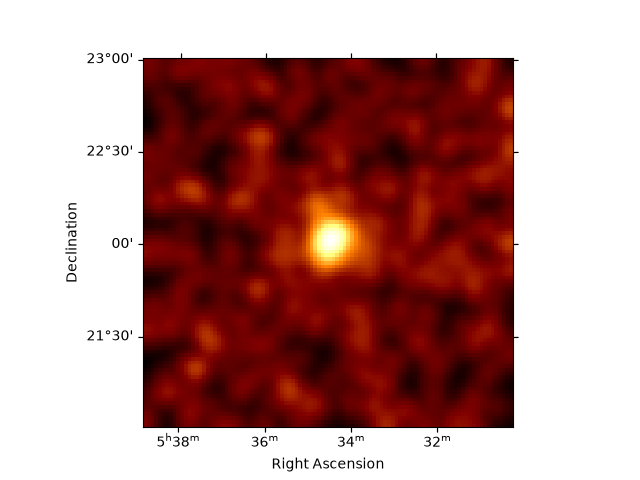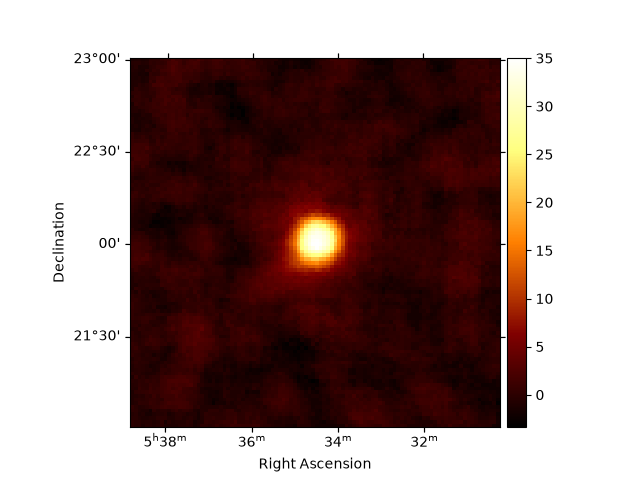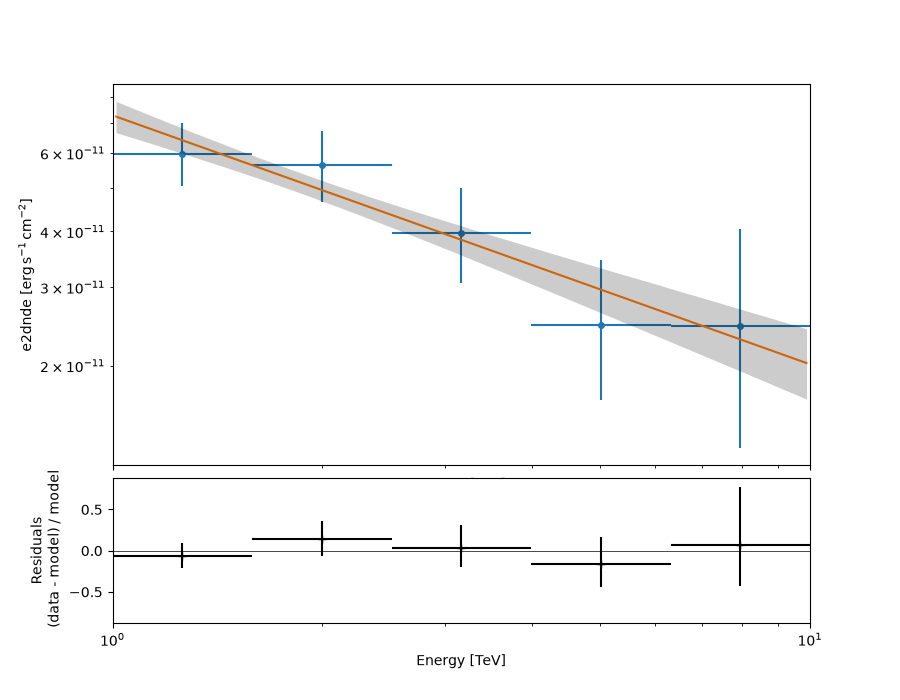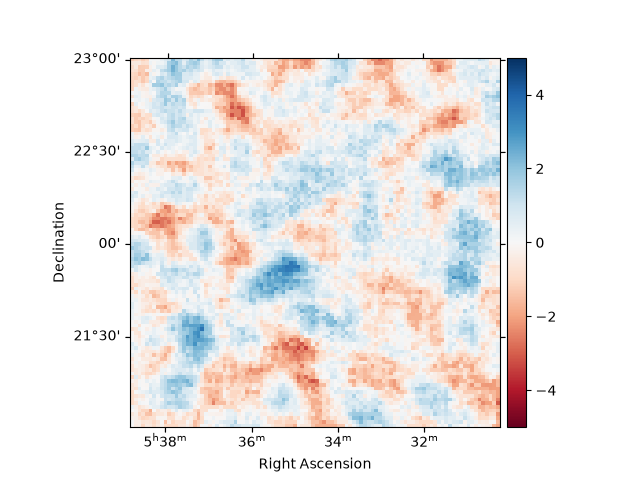# High level interface#

Introduction to 3D analysis using the Gammapy high level interface.

## Prerequisites#

• Understanding the gammapy data workflow, in particular what are DL3 events and instrument response functions (IRF).

## Context#

This notebook is an introduction to gammapy analysis using the high level interface.

Gammapy analysis consists in two main steps.

The first one is data reduction: user selected observations are reduced to a geometry defined by the user. It can be 1D (spectrum from a given extraction region) or 3D (with a sky projection and an energy axis). The resulting reduced data and instrument response functions (IRF) are called datasets in Gammapy.

The second step consists in setting a physical model on the datasets and fitting it to obtain relevant physical information.

Objective: Create a 3D dataset of the Crab using the H.E.S.S. DL3 data release 1 and perform a simple model fitting of the Crab nebula.

## Proposed approach#

This notebook uses the high level `Analysis` class to orchestrate data reduction. In its current state, `Analysis` supports the standard analysis cases of joint or stacked 3D and 1D analyses. It is instantiated with an `AnalysisConfig` object that gives access to analysis parameters either directly or via a YAML config file.

To see what is happening under-the-hood and to get an idea of the internal API, a second notebook performs the same analysis without using the `Analysis` class.

In summary, we have to:

• Create an `AnalysisConfig` object and edit it to define the analysis configuration:

• Define what observations to use

• Define the geometry of the dataset (data and IRFs)

• Define the model we want to fit on the dataset.

• Instantiate a `Analysis` from this configuration and run the different analysis steps

• Observation selection

• Data reduction

• Model fitting

• Estimating flux points

Finally we will compare the results against a reference model.

## Setup#

```# %matplotlib inline
from pathlib import Path
from astropy import units as u
from gammapy.analysis import Analysis, AnalysisConfig
```

## Check setup#

```from gammapy.utils.check import check_tutorials_setup

check_tutorials_setup()
```
```System:

python_executable      : /home/runner/micromamba-root/envs/gammapy-dev/bin/python
python_version         : 3.8.13
machine                : x86_64
system                 : Linux

Gammapy package:

version                : 0.20.2.dev535+g4ab0530ce
path                   : /home/runner/work/gammapy-docs/gammapy-docs/gammapy/gammapy

Other packages:

numpy                  : 1.22.4
scipy                  : 1.9.1
astropy                : 5.1
regions                : 0.6
click                  : 8.1.3
yaml                   : 6.0
IPython                : 8.5.0
jupyterlab             : 3.4.7
matplotlib             : 3.6.0
pandas                 : 1.5.0
healpy                 : 1.16.1
iminuit                : 2.16.0
sherpa                 : 4.14.1
naima                  : 0.10.0
emcee                  : 3.1.2
corner                 : 2.2.1

Gammapy environment variables:

GAMMAPY_DATA           : /home/runner/work/gammapy-docs/gammapy-docs/gammapy-datasets
```

## Analysis configuration#

For configuration of the analysis we use the YAML data format. YAML is a machine readable serialisation format, that is also friendly for humans to read. In this tutorial we will write the configuration file just using Python strings, but of course the file can be created and modified with any text editor of your choice.

Here is what the configuration for our analysis looks like:

```config = AnalysisConfig()
# the AnalysisConfig gives access to the various parameters used from logging to reduced dataset geometries
print(config)
```
```AnalysisConfig

general:
log: {level: info, filename: null, filemode: null, format: null, datefmt: null}
outdir: .
n_jobs: 1
datasets_file: null
models_file: null
observations:
datastore: \$GAMMAPY_DATA/hess-dl3-dr1
obs_ids: []
obs_file: null
obs_cone: {frame: null, lon: null, lat: null, radius: null}
obs_time: {start: null, stop: null}
required_irf: [aeff, edisp, psf, bkg]
datasets:
type: 1d
stack: true
geom:
wcs:
skydir: {frame: null, lon: null, lat: null}
binsize: 0.02 deg
width: {width: 5.0 deg, height: 5.0 deg}
binsize_irf: 0.2 deg
selection: {offset_max: 2.5 deg}
axes:
energy: {min: 1.0 TeV, max: 10.0 TeV, nbins: 5}
energy_true: {min: 0.5 TeV, max: 20.0 TeV, nbins: 16}
map_selection: [counts, exposure, background, psf, edisp]
background:
method: null
exclusion: null
parameters: {}
methods: [aeff-default]
parameters: {}
on_region: {frame: null, lon: null, lat: null, radius: null}
containment_correction: true
fit:
fit_range: {min: null, max: null}
flux_points:
energy: {min: null, max: null, nbins: null}
source: source
parameters: {selection_optional: all}
excess_map:
parameters: {}
energy_edges: {min: null, max: null, nbins: null}
light_curve:
time_intervals: {start: null, stop: null}
energy_edges: {min: null, max: null, nbins: null}
source: source
parameters: {selection_optional: all}
```

### Setting the data to use#

We want to use Crab runs from the H.E.S.S. DL3-DR1. We define here the datastore and a cone search of observations pointing with 5 degrees of the Crab nebula. Parameters can be set directly or as a python dict.

PS: do not forget to setup your environment variable \$GAMMAPY_DATA to your local directory containing the H.E.S.S. DL3-DR1 as described in Quickstart Setup.

```# We define the datastore containing the data
config.observations.datastore = "\$GAMMAPY_DATA/hess-dl3-dr1"

# We define the cone search parameters
config.observations.obs_cone.frame = "icrs"
config.observations.obs_cone.lon = "83.633 deg"
config.observations.obs_cone.lat = "22.014 deg"
config.observations.obs_cone.radius = "5 deg"

# Equivalently we could have set parameters with a python dict
# config.observations.obs_cone = {"frame": "icrs", "lon": "83.633 deg", "lat": "22.014 deg", "radius": "5 deg"}
```

### Setting the reduced datasets geometry#

```# We want to perform a 3D analysis
config.datasets.type = "3d"
# We want to stack the data into a single reduced dataset
config.datasets.stack = True

# We fix the WCS geometry of the datasets
config.datasets.geom.wcs.skydir = {
"lon": "83.633 deg",
"lat": "22.014 deg",
"frame": "icrs",
}
config.datasets.geom.wcs.width = {"width": "2 deg", "height": "2 deg"}
config.datasets.geom.wcs.binsize = "0.02 deg"

# We now fix the energy axis for the counts map
config.datasets.geom.axes.energy.min = "1 TeV"
config.datasets.geom.axes.energy.max = "10 TeV"
config.datasets.geom.axes.energy.nbins = 10

# We now fix the energy axis for the IRF maps (exposure, etc)
config.datasets.geom.axes.energy_true.min = "0.5 TeV"
config.datasets.geom.axes.energy_true.max = "20 TeV"
config.datasets.geom.axes.energy_true.nbins = 20
```

### Setting the background normalization maker#

```config.datasets.background.method = "fov_background"
config.datasets.background.parameters = {"method": "scale"}
```

### Setting the exclusion mask#

In order to properly adjust the background normalisation on regions without gamma-ray signal, one needs to define an exclusion mask for the background normalisation. For this tutorial, we use the following one `\$GAMMAPY_DATA/joint-crab/exclusion/exclusion_mask_crab.fits.gz`

```config.datasets.background.exclusion = (
)
```

### Setting modeling and fitting parameters#

`Analysis` can perform a few modeling and fitting tasks besides data reduction. Parameters have then to be passed to the configuration object.

Here we define the energy range on which to perform the fit. We also set the energy edges used for flux point computation as well as the correlation radius to compute excess and significance maps.

```config.fit.fit_range.min = 1 * u.TeV
config.fit.fit_range.max = 10 * u.TeV
config.flux_points.energy = {"min": "1 TeV", "max": "10 TeV", "nbins": 4}
config.excess_map.correlation_radius = 0.1 * u.deg
```

We’re all set. But before we go on let’s see how to save or import `AnalysisConfig` objects though YAML files.

### Using YAML configuration files#

One can export/import the `AnalysisConfig` to/from a YAML file.

```config.write("config.yaml", overwrite=True)

print(config)
```
```AnalysisConfig

general:
log: {level: info, filename: null, filemode: null, format: null, datefmt: null}
outdir: .
n_jobs: 1
datasets_file: null
models_file: null
observations:
datastore: \$GAMMAPY_DATA/hess-dl3-dr1
obs_ids: []
obs_file: null
obs_cone: {frame: icrs, lon: 83.633 deg, lat: 22.014 deg, radius: 5.0 deg}
obs_time: {start: null, stop: null}
required_irf: [aeff, edisp, psf, bkg]
datasets:
type: 3d
stack: true
geom:
wcs:
skydir: {frame: icrs, lon: 83.633 deg, lat: 22.014 deg}
binsize: 0.02 deg
width: {width: 2.0 deg, height: 2.0 deg}
binsize_irf: 0.2 deg
selection: {offset_max: 2.5 deg}
axes:
energy: {min: 1.0 TeV, max: 10.0 TeV, nbins: 10}
energy_true: {min: 0.5 TeV, max: 20.0 TeV, nbins: 20}
map_selection: [counts, exposure, background, psf, edisp]
background:
method: fov_background
parameters: {method: scale}
methods: [aeff-default]
parameters: {}
on_region: {frame: null, lon: null, lat: null, radius: null}
containment_correction: true
fit:
fit_range: {min: 1.0 TeV, max: 10.0 TeV}
flux_points:
energy: {min: 1.0 TeV, max: 10.0 TeV, nbins: 4}
source: source
parameters: {selection_optional: all}
excess_map:
parameters: {}
energy_edges: {min: null, max: null, nbins: null}
light_curve:
time_intervals: {start: null, stop: null}
energy_edges: {min: null, max: null, nbins: null}
source: source
parameters: {selection_optional: all}
```

## Running the analysis#

We first create an `Analysis` object from our configuration.

```analysis = Analysis(config)
```
```Setting logging config: {'level': 'INFO', 'filename': None, 'filemode': None, 'format': None, 'datefmt': None}
```

### Observation selection#

We can directly select and load the observations from disk using `get_observations()`:

```analysis.get_observations()
```
```Fetching observations.
Observations selected: 4 out of 4.
Number of selected observations: 4
```

The observations are now available on the `Analysis` object. The selection corresponds to the following ids:

```analysis.observations.ids
```

To see how to explore observations, please refer to the following notebook: CTA with Gammapy or HESS with Gammapy

## Data reduction#

Now we proceed to the data reduction. In the config file we have chosen a WCS map geometry, energy axis and decided to stack the maps. We can run the reduction using `get_datasets()`:

```analysis.get_datasets()
```
```Creating reference dataset and makers.
Creating the background Maker.
Start the data reduction loop.
Computing dataset for observation 23523
Running MapDatasetMaker
Running FoVBackgroundMaker
Computing dataset for observation 23526
Running MapDatasetMaker
Running FoVBackgroundMaker
Computing dataset for observation 23559
Running MapDatasetMaker
Running FoVBackgroundMaker
Computing dataset for observation 23592
Running MapDatasetMaker
Running FoVBackgroundMaker
```

As we have chosen to stack the data, there is finally one dataset contained which we can print:

```print(analysis.datasets["stacked"])
```
```MapDataset
----------

Name                            : stacked

Total counts                    : 2485
Total background counts         : 1997.49
Total excess counts             : 487.51

Predicted counts                : 1997.49
Predicted background counts     : 1997.49
Predicted excess counts         : nan

Exposure min                    : 2.73e+08 m2 s
Exposure max                    : 3.52e+09 m2 s

Number of total bins            : 100000
Number of fit bins              : 100000

Fit statistic type              : cash
Fit statistic value (-2 log(L)) : nan

Number of models                : 0
Number of parameters            : 0
Number of free parameters       : 0
```

As you can see the dataset comes with a predefined background model out of the data reduction, but no source model has been set yet.

The counts, exposure and background model maps are directly available on the dataset and can be printed and plotted:

```counts = analysis.datasets["stacked"].counts
counts.smooth("0.05 deg").plot_interactive()
``````interactive(children=(SelectionSlider(continuous_update=False, description='Select energy:', layout=Layout(width='50%'), options=('1.00 TeV - 1.26 TeV', '1.26 TeV - 1.58 TeV', '1.58 TeV - 2.00 TeV', '2.00 TeV - 2.51 TeV', '2.51 TeV - 3.16 TeV', '3.16 TeV - 3.98 TeV', '3.98 TeV - 5.01 TeV', '5.01 TeV - 6.31 TeV', '6.31 TeV - 7.94 TeV', '7.94 TeV - 10.0 TeV'), style=SliderStyle(description_width='initial'), value='1.00 TeV - 1.26 TeV'), RadioButtons(description='Select stretch:', index=1, options=('linear', 'sqrt', 'log'), style=DescriptionStyle(description_width='initial'), value='sqrt'), Output()), _dom_classes=('widget-interact',))
```

We can also compute the map of the sqrt_ts (significance) of the excess counts above the background. The correlation radius to sum counts is defined in the config file.

```analysis.get_excess_map()
``````Computing excess maps.
```

## Save dataset to disk#

It is common to run the preparation step independent of the likelihood fit, because often the preparation of maps, PSF and energy dispersion is slow if you have a lot of data. We first create a folder:

```path = Path("analysis_1")
path.mkdir(exist_ok=True)
```

And then write the maps and IRFs to disk by calling the dedicated `write` method:

```filename = path / "crab-stacked-dataset.fits.gz"
analysis.datasets.write(filename, overwrite=True)
```

## Model fitting#

Now we define a model to be fitted to the dataset. Here we use its YAML definition to load it:

```model_config = """
components:
- name: crab
type: SkyModel
spatial:
type: PointSpatialModel
frame: icrs
parameters:
- name: lon_0
value: 83.63
unit: deg
- name: lat_0
value: 22.014
unit: deg
spectral:
type: PowerLawSpectralModel
parameters:
- name: amplitude
value: 1.0e-12
unit: cm-2 s-1 TeV-1
- name: index
value: 2.0
unit: ''
- name: reference
value: 1.0
unit: TeV
frozen: true
"""
```

Now we set the model on the analysis object:

```analysis.set_models(model_config)
```
```Reading model.
Models

Component 0: SkyModel

Name                      : crab
Datasets names            : None
Spectral model type       : PowerLawSpectralModel
Spatial  model type       : PointSpatialModel
Temporal model type       :
Parameters:
index                         :      2.000   +/-    0.00
amplitude                     :   1.00e-12   +/- 0.0e+00 1 / (cm2 s TeV)
reference             (frozen):      1.000       TeV
lon_0                         :     83.630   +/-    0.00 deg
lat_0                         :     22.014   +/-    0.00 deg

Component 1: FoVBackgroundModel

Name                      : stacked-bkg
Datasets names            : ['stacked']
Spectral model type       : PowerLawNormSpectralModel
Parameters:
norm                          :      1.000   +/-    0.00
tilt                  (frozen):      0.000
reference             (frozen):      1.000       TeV
```

Finally we run the fit:

```analysis.run_fit()

print(analysis.fit_result)
```
```Fitting datasets.
OptimizeResult

backend    : minuit
success    : True
message    : Optimization terminated successfully.
nfev       : 263
total stat : 19991.99

CovarianceResult

backend    : minuit
method     : hesse
success    : True
message    : Hesse terminated successfully.

OptimizeResult

backend    : minuit
success    : True
message    : Optimization terminated successfully.
nfev       : 263
total stat : 19991.99

CovarianceResult

backend    : minuit
method     : hesse
success    : True
message    : Hesse terminated successfully.
```

This is how we can write the model back to file again:

```filename = path / "model-best-fit.yaml"
analysis.models.write(filename, overwrite=True)

with filename.open("r") as f:
```
```components:
-   name: crab
type: SkyModel
spectral:
type: PowerLawSpectralModel
parameters:
-   name: index
value: 2.5562210791489153
error: 0.10315678645288112
-   name: amplitude
value: 4.5503636370123015e-11
unit: cm-2 s-1 TeV-1
error: 3.73323343455769e-12
-   name: reference
value: 1.0
unit: TeV
frozen: true
spatial:
type: PointSpatialModel
frame: icrs
parameters:
-   name: lon_0
value: 83.61982254758775
unit: deg
error: 0.0031464003067912206
-   name: lat_0
value: 22.024551464549514
unit: deg
error: 0.0029614977213814012
-   type: FoVBackgroundModel
datasets_names:
- stacked
spectral:
type: PowerLawNormSpectralModel
parameters:
-   name: norm
value: 0.9864653706689446
error: 0.023473086803459966
-   name: tilt
value: 0.0
frozen: true
-   name: reference
value: 1.0
unit: TeV
frozen: true
covariance: model-best-fit_covariance.dat
```

### Flux points#

```analysis.config.flux_points.source = "crab"
# Example showing how to change the FluxPointsEstimator parameters:
analysis.config.flux_points.energy.nbins = 5
config_dict = {
"selection_optional": "all",
"n_sigma": 2,  # Number of sigma to use for asymmetric error computation
"n_sigma_ul": 3,  # Number of sigma to use for upper limit computation
}
analysis.config.flux_points.parameters = config_dict

analysis.get_flux_points()

# Example showing how to change just before plotting the threshold on the signal significance
# (points vs upper limits), even if this has no effect with this data set.
fp = analysis.flux_points.data
fp.sqrt_ts_threshold_ul = 5
ax_sed, ax_residuals = analysis.flux_points.plot_fit()
``````Calculating flux points.
Reoptimize = False ignored for iminuit backend
Reoptimize = False ignored for iminuit backend
Reoptimize = False ignored for iminuit backend
Reoptimize = False ignored for iminuit backend
Reoptimize = False ignored for iminuit backend
Reoptimize = False ignored for iminuit backend
Reoptimize = False ignored for iminuit backend
Reoptimize = False ignored for iminuit backend
Reoptimize = False ignored for iminuit backend
Reoptimize = False ignored for iminuit backend

e_ref                 dnde          ...      sqrt_ts
TeV            1 / (cm2 s TeV)     ...
------------------ ---------------------- ... ------------------
1.2589254117941673  2.356608777288396e-11 ...  24.26513173801644
1.9952623149688797  8.856744568527033e-12 ... 22.402060413130474
3.1622776601683795 2.4889099682075633e-12 ... 16.767169984811108
5.011872336272723  6.151508387857421e-13 ...  11.90598020946889
7.943282347242818 2.4384523563139535e-13 ...   8.29407821611322
```

The flux points can be exported to a fits table following the format defined here

```filename = path / "flux-points.fits"
analysis.flux_points.write(filename, overwrite=True)
```

To check the fit is correct, we compute the map of the sqrt_ts of the excess counts above the current model.

```analysis.get_excess_map()
analysis.excess_map["sqrt_ts"].plot(add_cbar=True, cmap="RdBu", vmin=-5, vmax=5)
``````Computing excess maps.
```

## What’s next#

You can look at the same analysis without the high level interface in analysis_2

You can see how to perform a 1D spectral analysis of the same data in spectral analysis

Total running time of the script: ( 0 minutes 28.054 seconds)

Gallery generated by Sphinx-Gallery